# Data Driven Christmas Card Animation with Voronoi Tiles

## Dec 21, 2016 · 1543 words · 8 minutes read R design ggforce tweenr

I recently published a bit of self-promotion in the form of an animation based on the new `geom_voronoi_tile()` and `geom_voronoi_segment()` functions in `ggforce`:

This prompted someone to ask for the source code and I off-handedly said I would make a blog post about. So here we are, but I’m not going to show you how I made that animation (sorry). Instead I’m going to show you how to make your very own animated Christmas card using `ggplot2`, `ggforce`, and `tweenr` (as well as `mgcv` and `deldir` for some computations).

Let’s set the scene: we are going to do a cyclic animation switching between a heart, the word “merry”, and the word “x-mas”. Each shape will be made up of voronoi tiles with the colour of the tile defining the shape. The shapes has been created in Illustrator and exported to a json representation using a script.

Let’s start by importing the shapes and do a sanity check:

``````library(jsonlite)
library(ggplot2)
layer_names <- greet\$layers\$name
heart <- greet\$layers\$paths[[which(layer_names == 'Heart')]]\$points
merry <- greet\$layers\$paths[[which(layer_names == 'Merry')]]\$points
x_mas <- greet\$layers\$paths[[which(layer_names == 'X-MAS')]]\$points
range <- greet\$layers\$paths[[which(layer_names == 'back')]]\$points[]

ggplot(as.data.frame(heart[])) +
geom_polygon(aes(V1, V2))``````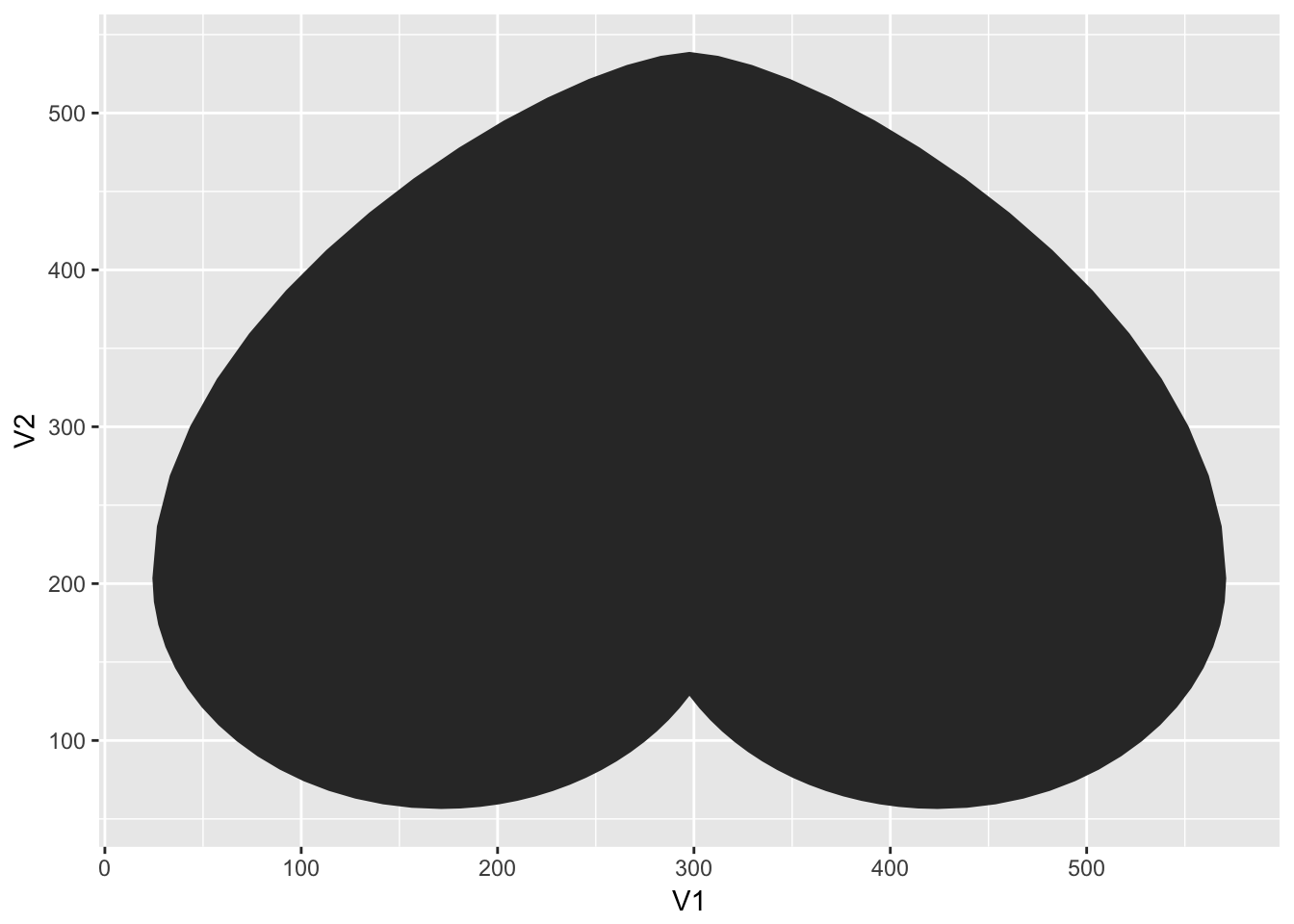That seems sort-of right. The shape is on its head since Illustrator measures its coordinates from the top-left corner and down and not from the bottom-left corner and up. Well simply use `scale_y_reverse()` later to circumvent this.

Now that we have our shapes it is time to draw them with points. To do this we’ll sample 25.000 points within the plot area and check which ones falls into the different shapes. There are many ways to test whether a point lies inside a shape - I’ll use the `in.out()` function from the `mgcv` package.

``````library(mgcv)
heart_points <- data.frame(
x = runif(25000, min = min(range[,1]), max = max(range[, 1])),
y = runif(25000, min = min(range[,2]), max = max(range[, 2])),
colour = 'green',
stringsAsFactors = FALSE
)
heart_points\$colour[
in.out(
do.call(rbind, lapply(heart, rbind, c(NA, NA))),
cbind(heart_points\$x, heart_points\$y)
)
] <- 'red'

merry_points <- data.frame(
x = runif(25000, min = min(range[,1]), max = max(range[, 1])),
y = runif(25000, min = min(range[,2]), max = max(range[, 2])),
colour = 'red',
stringsAsFactors = FALSE
)
merry_points\$colour[
in.out(
do.call(rbind, lapply(merry, rbind, c(NA, NA))),
cbind(merry_points\$x, merry_points\$y)
)
] <- 'green'

xmas_points <- data.frame(
x = runif(25000, min = min(range[,1]), max = max(range[, 1])),
y = runif(25000, min = min(range[,2]), max = max(range[, 2])),
colour = 'red',
stringsAsFactors = FALSE
)
xmas_points\$colour[
in.out(
do.call(rbind, lapply(x_mas, rbind, c(NA, NA))),
cbind(xmas_points\$x, xmas_points\$y)
)
] <- 'green'

ggplot(merry_points) +
geom_point(aes(x, y, colour = colour))``````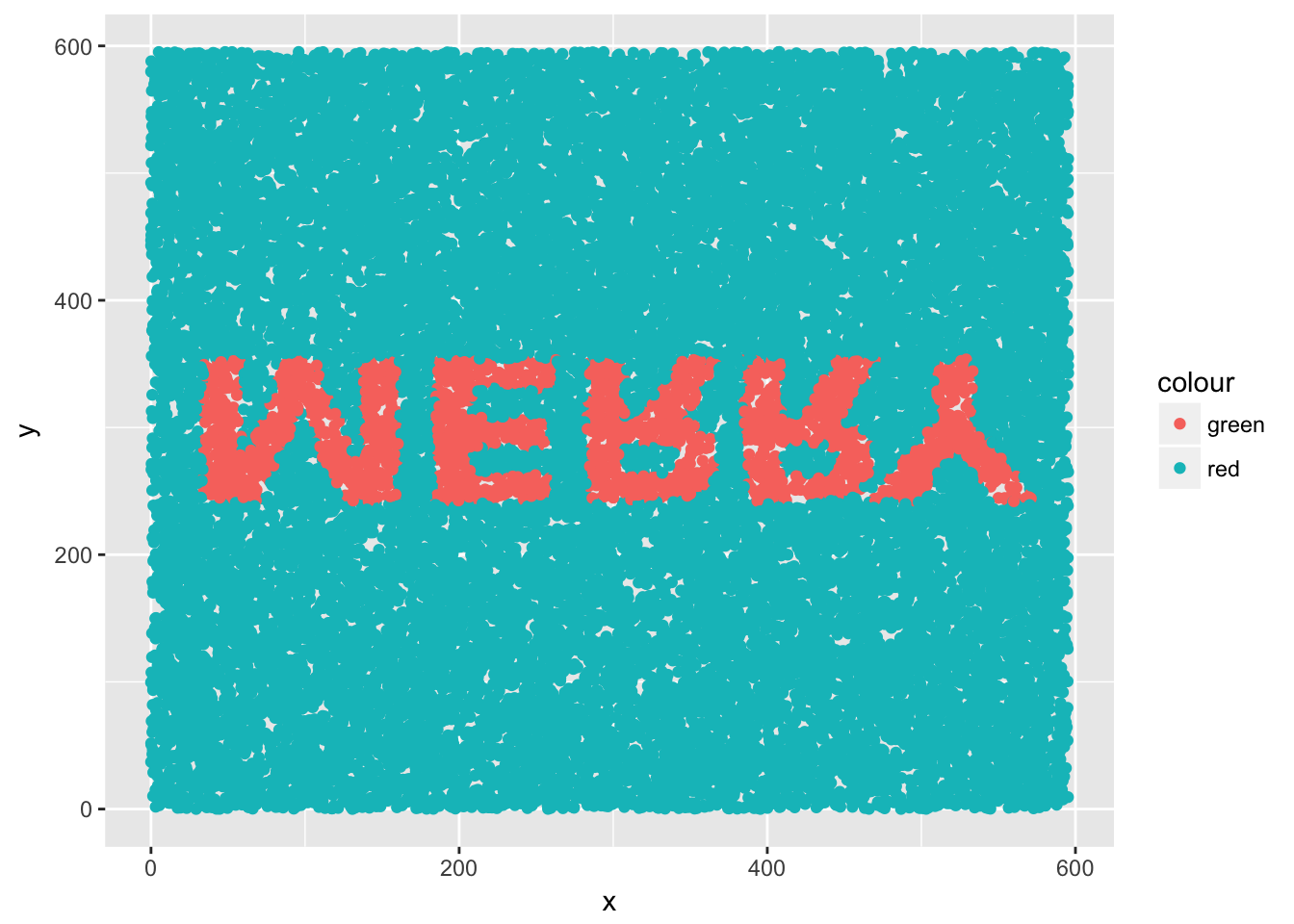We could call this a day and continue with all those points, but we wont. First, because voronoi tessellation (as implemented in `deldir`) does not scale well to these number of points, and second, because the tiles would be so small that they are almost indistinguishable from each other.

To fix this we are going to remove a lot of the points that do not help in defining the shapes. In order to determine which points are safe to remove we are going to calculate the delaunay triangulation (the “inverse” of a voronoi tesselation) and find out which points only have neighbors of the same colour. We are going to remove as many random point so well end up with 1.000 points of each colour:

``````library(deldir)
library(ggforce)

heart_vor <- deldir(heart_points\$x, heart_points\$y)
heart_con <- split(c(heart_points\$colour[heart_vor\$delsgs\$ind2],
heart_points\$colour[heart_vor\$delsgs\$ind1]),
c(heart_vor\$delsgs\$ind1, heart_vor\$delsgs\$ind2))
heart_boring <- lengths(lapply(heart_con, unique)) == 1
heart_remove <- c(
sample(which(heart_boring & heart_points\$colour == 'red'),
sum(heart_boring & heart_points\$colour == 'red') -
(1000 - sum(!heart_boring & heart_points\$colour == 'red'))),
sample(which(heart_boring & heart_points\$colour == 'green'),
sum(heart_boring & heart_points\$colour == 'green') -
(1000 - sum(!heart_boring & heart_points\$colour == 'green')))
)
heart_points <- heart_points[-heart_remove, ]

merry_vor <- deldir(merry_points\$x, merry_points\$y)
merry_con <- split(c(merry_points\$colour[merry_vor\$delsgs\$ind2],
merry_points\$colour[merry_vor\$delsgs\$ind1]),
c(merry_vor\$delsgs\$ind1, merry_vor\$delsgs\$ind2))
merry_boring <- lengths(lapply(merry_con, unique)) == 1
merry_remove <- c(
sample(which(merry_boring & merry_points\$colour == 'red'),
sum(merry_boring & merry_points\$colour == 'red') -
(1000 - sum(!merry_boring & merry_points\$colour == 'red'))),
sample(which(merry_boring & merry_points\$colour == 'green'),
sum(merry_boring & merry_points\$colour == 'green') -
(1000 - sum(!merry_boring & merry_points\$colour == 'green')))
)
merry_points <- merry_points[-merry_remove, ]

xmas_vor <- deldir(xmas_points\$x, xmas_points\$y)
xmas_con <- split(c(xmas_points\$colour[xmas_vor\$delsgs\$ind2],
xmas_points\$colour[xmas_vor\$delsgs\$ind1]),
c(xmas_vor\$delsgs\$ind1, xmas_vor\$delsgs\$ind2))
xmas_boring <- lengths(lapply(xmas_con, unique)) == 1
xmas_remove <- c(
sample(which(xmas_boring & xmas_points\$colour == 'red'),
sum(xmas_boring & xmas_points\$colour == 'red') -
(1000 - sum(!xmas_boring & xmas_points\$colour == 'red'))),
sample(which(xmas_boring & xmas_points\$colour == 'green'),
sum(xmas_boring & xmas_points\$colour == 'green') -
(1000 - sum(!xmas_boring & xmas_points\$colour == 'green')))
)
xmas_points <- xmas_points[-xmas_remove, ]

ggplot(xmas_points) +
geom_voronoi_tile(aes(x, y, fill = colour), colour = 'black')``````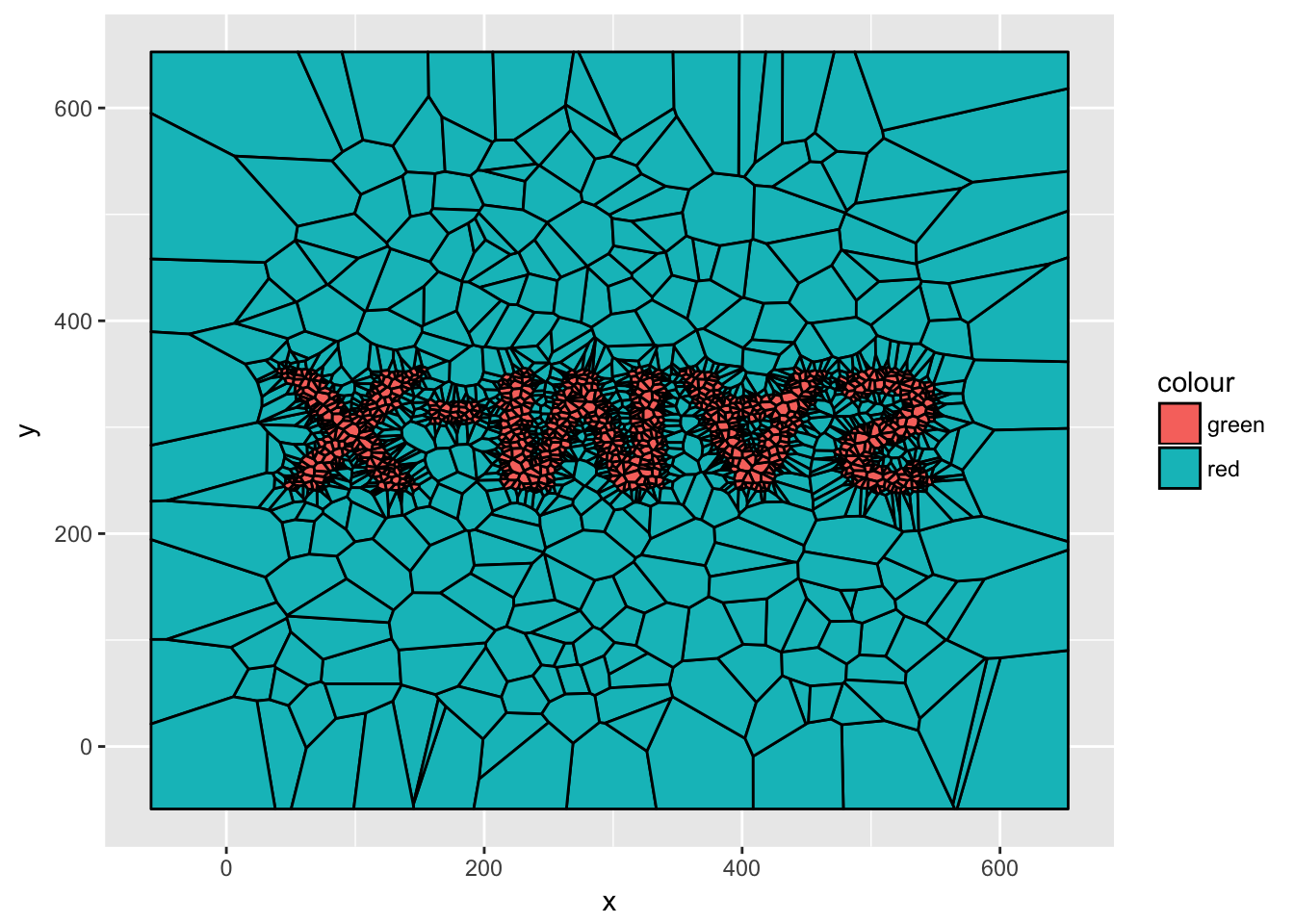Now we’re getting somewhere!

The next step is to match the points from each stage together so we know which one moves where. For the transition between the heart and the text I’m fine with it being random, but for the transition between “merry” and “x-mas” I want it to seem as smooth as possible. This requires that each point in `merry_points` get matched to its nearest neighbor of equal colour in the `xmas_points` data.frame. As some points might compete for the nearest neighbor some will have to settle for neighbors a bit farther away. While it might be possible to optimise the choice of neighbor we are just going to go through each in turn so the points occuring first in the data.frame will get first pick:

``````heart_points <- heart_points[order(heart_points\$colour), ]
heart_points\$id <- seq_len(nrow(heart_points))
merry_points <- merry_points[order(merry_points\$colour), ]
merry_points\$id <- seq_len(nrow(merry_points))
xmas_points <- xmas_points[order(xmas_points\$colour), ]

eu_dist <- function(x, y) {
ifelse(
merry_points\$colour[x] != xmas_points\$colour[y],
1e5,
sqrt((merry_points\$x[x] - xmas_points\$x[y])^2 +
(merry_points\$y[x] - xmas_points\$y[y])^2)
)
}
distance <- outer(seq_len(nrow(merry_points)),
seq_len(nrow(merry_points)),
eu_dist
)

pair <- Reduce(function(l, r) {
pair_order <- order(distance[r, ])
c(l, pair_order[which(!pair_order %in% l)])
}, seq_len(nrow(merry_points)), init = integer())

xmas_points\$id[pair] <- seq_len(nrow(xmas_points))
xmas_points <- xmas_points[order(xmas_points\$id), ]``````

Now each data.frame is lined up so the point encoded in row 1 is the same across all. The last bit of work we need to do is to interpolate the transition between each stage. This could be done in one line of code with `tweenr` using `tween_states()` but I dont want all points to start and end their movements at the same time. Thus we need to do something a bit more custom:

``````library(tweenr)
tween_points <- function(from, to, length, stagger) {
leave <- sample(seq_len(stagger), nrow(from), replace = TRUE)
arive <- sample(seq_len(stagger), nrow(from), replace = TRUE)
x <- tween_t(Map(function(.f, .t) c(.f, .t), .f = from\$x, .t = to\$x),
length - leave - arive)
y <- tween_t(Map(function(.f, .t) c(.f, .t), .f = from\$y, .t = to\$y),
length - leave - arive)
points <- Map(function(x, y, l, a) {
data.frame(x = x, y = y)[c(rep(1, l), seq_along(x), rep(length(x), a)), ]
}, x = x, y = y, l = leave, a = arive)
points <- do.call(rbind, points)
points\$frame <- rep(seq_len(length), nrow(from))
cbind(
points,
from[rep(seq_len(nrow(from)), each = length), !names(from) %in% c('x', 'y')])
}``````

This function takes two data.frames along with the total transition length and a `stagger` value that encodes the maximum offset in time each transition can have in terms of starting and ending. It then interpolates the `x` and `y` columns between the two data.frames, adding a `frame` column to the end result.

Let’s put it to good use. Our animation will take 12 seconds at a frame rate of 24 fps making a total of 288 frames and 96 frames for each stage along with the transition to it. We’ll split it up so that each stage is hold for 36 frames, ending up with 60 frames for each transition. We’ll allow the start and end time to vary with 20 frames each:

``````n_frames_still <- 36
n_frames_trans <- 60
n_frames_stagger <- 20
n_frames_stage <- 96

heart_to_merry <- tween_points(heart_points,
merry_points,
n_frames_trans,
n_frames_stagger)
merry_to_xmas <- tween_points(merry_points,
xmas_points,
n_frames_trans,
n_frames_stagger)
xmas_to_heart <- tween_points(xmas_points,
heart_points,
n_frames_trans,
n_frames_stagger)``````

In addition to this we need to repeat each stage 36 times and offset the frame count. In the end we’ll combine everything into one data.frame:

``````heart_still <- heart_points[rep(seq_len(nrow(heart_points)), n_frames_still), ]
heart_still\$frame <- rep(seq_len(n_frames_still), each = nrow(heart_points))
heart_to_merry\$frame <- heart_to_merry\$frame + n_frames_still

merry_still <- merry_points[rep(seq_len(nrow(merry_points)), n_frames_still), ]
merry_still\$frame <- rep(seq_len(n_frames_still), each = nrow(merry_points)) + n_frames_stage
merry_to_xmas\$frame <- merry_to_xmas\$frame + n_frames_stage + n_frames_still

xmas_still <- xmas_points[rep(seq_len(nrow(xmas_points)), n_frames_still), ]
xmas_still\$frame <- rep(seq_len(n_frames_still), each = nrow(xmas_points)) + 2*n_frames_stage
xmas_to_heart\$frame <- xmas_to_heart\$frame + 2*n_frames_stage + n_frames_still

frames <- rbind(
heart_still,
heart_to_merry,
merry_still,
merry_to_xmas,
xmas_still,
xmas_to_heart
)``````

We now have everything in place to create our animation - we just need to define the plotting function (take note that we specify the bounding rectangle as the range of our data might change between frames):

``````christmas_card <- function(data) {
bound <- c(range(range[,1]), range(-range[,2]))
ggplot(data, aes(x = x, y = y)) +
geom_voronoi_tile(aes(fill = colour), bound = bound) +
geom_voronoi_segment(alpha = 0.3, bound = bound) +
scale_y_reverse() +
scale_fill_manual(values = c('forestgreen', 'firebrick')) +
labs(caption = 'www.data-imaginist.com') +
coord_fixed(expand = FALSE) +
theme_void() +
theme(legend.position = 'none')
}
plot(christmas_card(heart_points))``````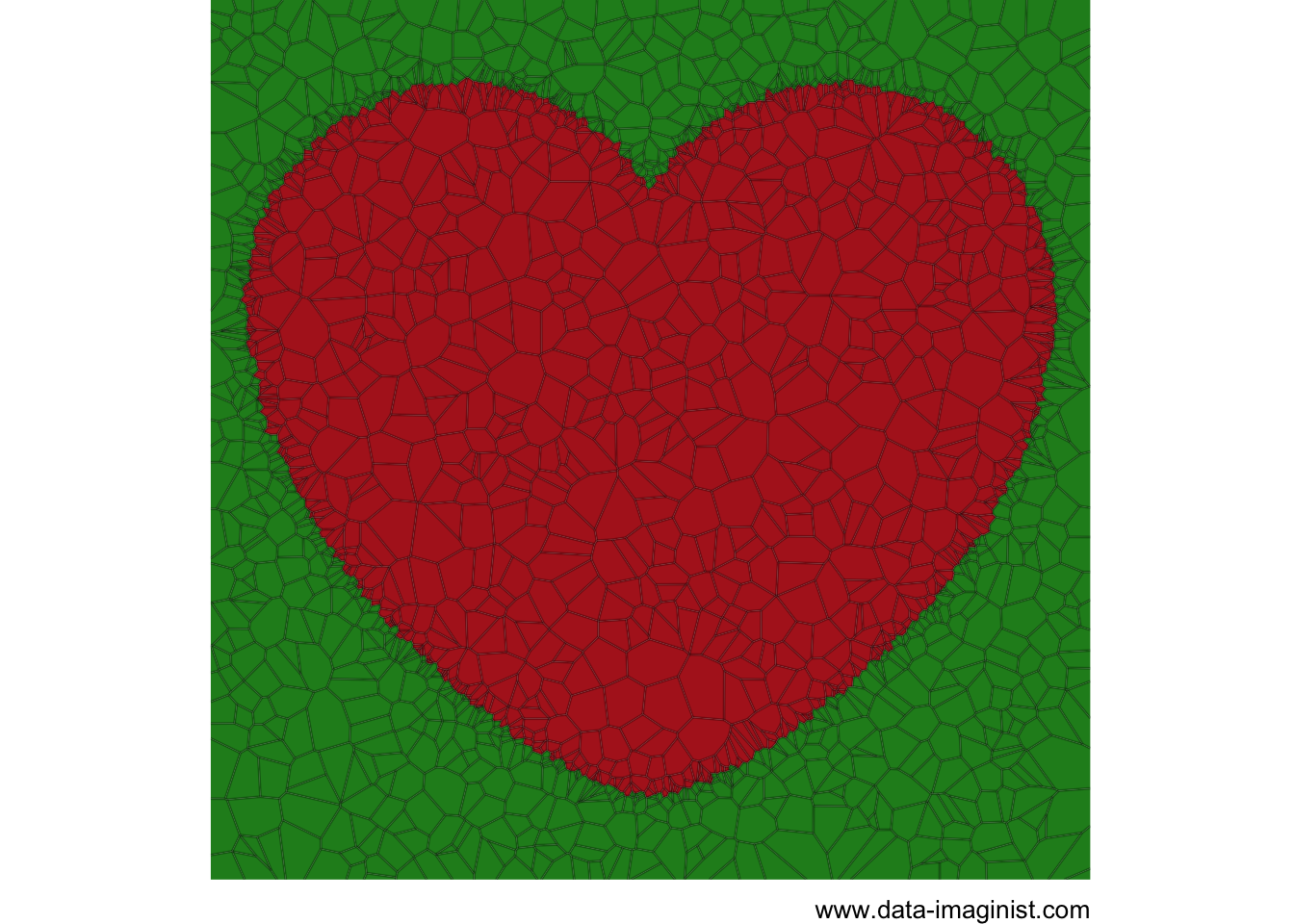Now it is just a matter of looping over each frame and plotting that data. Normally `gganimate` would be useful for this but it does not always play nice when data is heavily transformed by the stat function (as is the case with `geom_voronoi_tile()` and `geom_voronoi_segment()`). We’ll just do it the oldschool way (if you’re running this yourself wrap the following expression in `animation::saveGIF()`):

``````for (i in seq_len(max(frames\$frame))) {
data <- frames[frames\$frame == i, ]
plot(christmas_card(data))
}``````
Merry Christmas!